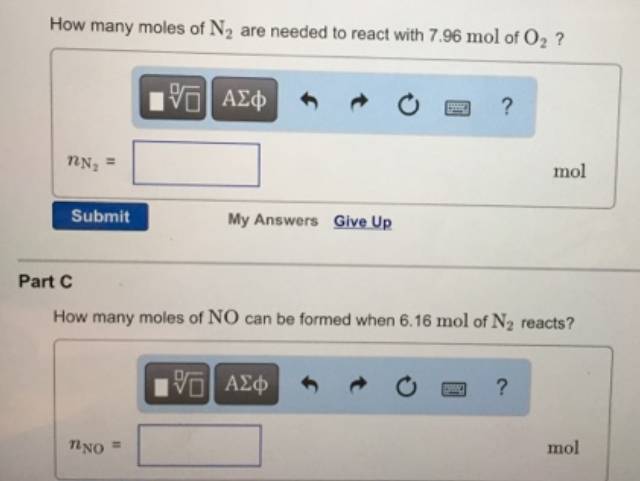Chemistry Stoichiometry Solution: How many moles of N2 are needed to react with 7.96...

# Solution: How many moles of N2 are needed to react with 7.96 mol of O2? How many moles of NO can be formed when 6.16 mol of N2 reacts?

Problem

How many moles of N2 are needed to react with 7.96 mol of O2?

How many moles of NO can be formed when 6.16 mol of N2 reacts?View Complete Written Solution